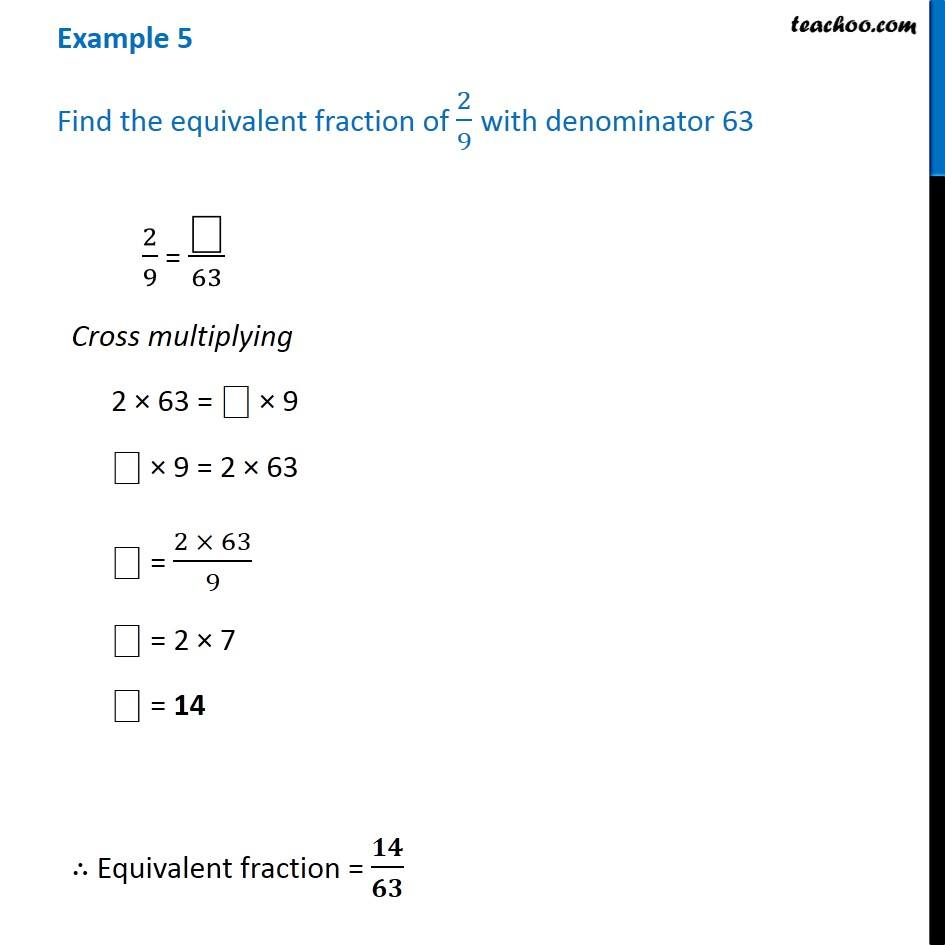Equivalent Fractions

Chapter 7 Class 6 Fractions
Concept wiseIntroducing your new favourite teacher - Teachoo Black, at only ₹83 per month

### Transcript

Example 5 Find the equivalent fraction of 2/9 with denominator 63 2/9 = "⎕" /63 Cross multiplying 2 × 63 = "⎕" × 9 "⎕" × 9 = 2 × 63 "⎕" = (2 × 63)/9 "⎕" = 2 × 7 "⎕" = 14 ∴ Equivalent fraction = 𝟏𝟒/𝟔𝟑